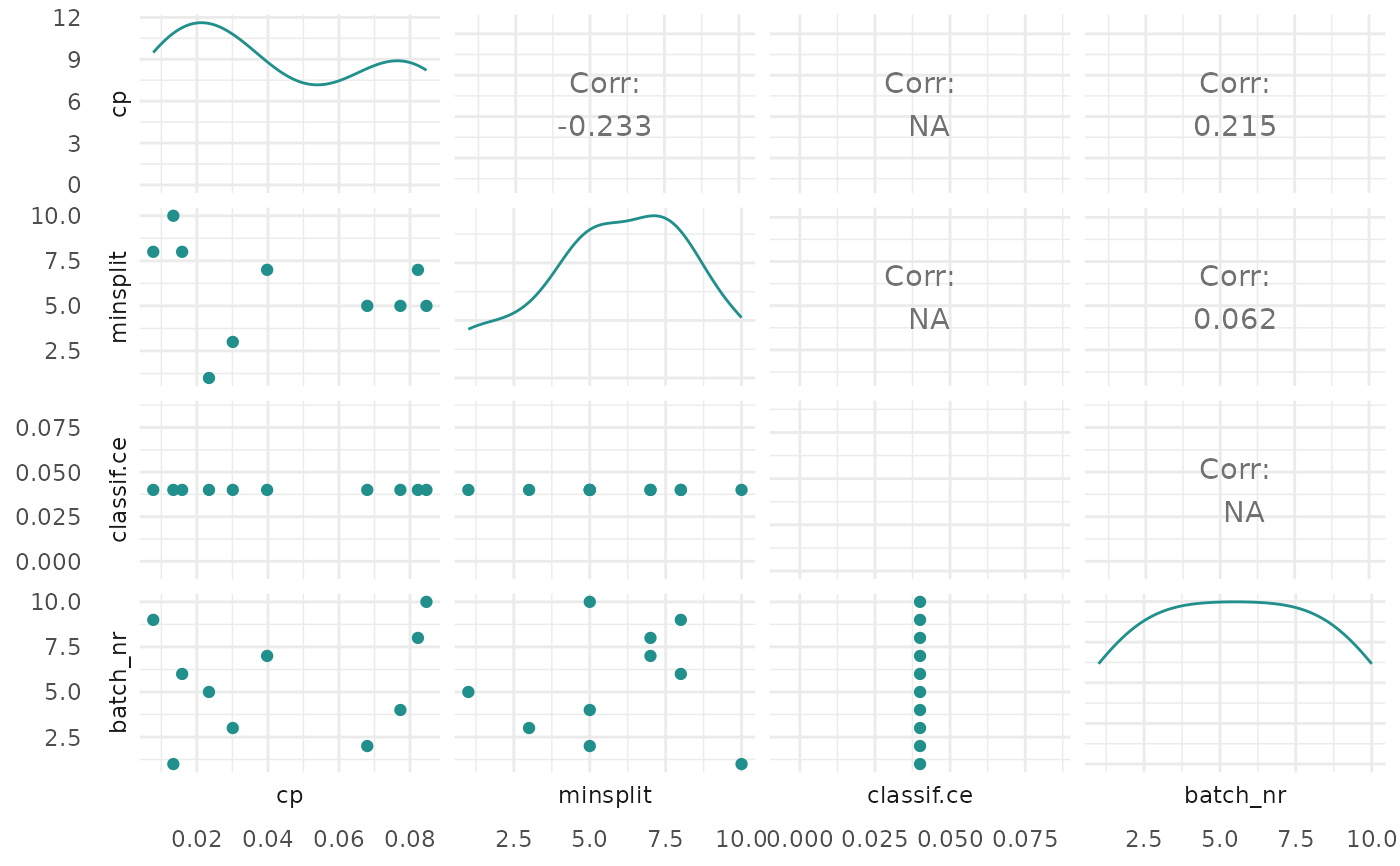Visualizations for mlr3tuning::TuningInstanceSingleCrit. The argument type controls what kind of plot is drawn. Possible choices are:

• "marginal" (default): Scatter plots of x versus y. The color of the points shows the batch number.

• "performance": Scatter plots of batch number versus y

• "parameter": Scatter plots of batch number versus input. The color of the points shows the y values.

• "parallel": Parallel coordinates plot. hyperparameters are rescaled by (x - mean(x)) / sd(x).

• "points": Scatter plot of two x dimensions versus. The color of the points shows the y values.

• "surface": Surface plot of two x dimensions versus y values. The y values are interpolated with the supplied mlr3::Learner.

• "pairs": Plots all x and y values against each other.

• "incumbent": Plots the incumbent versus the number of configurations.

## Usage

# S3 method for TuningInstanceSingleCrit
autoplot(
object,
type = "marginal",
cols_x = NULL,
trafo = FALSE,
learner = mlr3::lrn("regr.ranger"),
grid_resolution = 100,
theme = theme_minimal(),
...
)

## Arguments

object
type

(character(1)):
Type of the plot. See description.

cols_x

(character())
Column names of hyperparameters. By default, all untransformed hyperparameters are plotted. Transformed hyperparameters are prefixed with x_domain_.

trafo

(logical(1))
If FALSE (default), the untransformed hyperparameters are plotted. If TRUE, the transformed hyperparameters are plotted.

learner

(mlr3::Learner)
Regression learner used to interpolate the data of the surface plot.

grid_resolution

(numeric())
Resolution of the surface plot.

theme

(ggplot2::theme())
The ggplot2::theme_minimal() is applied by default to all plots.

...

(ignored).

## Value

ggplot2::ggplot().

## Examples

if (requireNamespace("mlr3tuning") && requireNamespace("patchwork")) {
library(mlr3tuning)

learner = lrn("classif.rpart")
learner$param_set$values$cp = to_tune(0.001, 0.1) learner$param_set$values$minsplit = to_tune(1, 10)

instance = TuningInstanceSingleCrit$new( task = tsk("iris"), learner = learner, resampling = rsmp("holdout"), measure = msr("classif.ce"), terminator = trm("evals", n_evals = 10)) tuner = tnr("random_search") tuner$optimize(instance)

# plot performance versus batch number
autoplot(instance, type = "performance")

# plot cp values versus performance
autoplot(instance, type = "marginal", cols_x = "cp")

# plot transformed parameter values versus batch number
autoplot(instance, type = "parameter", trafo = TRUE)

# plot parallel coordinates plot
autoplot(instance, type = "parallel")

# plot pairs
autoplot(instance, type = "pairs")
}